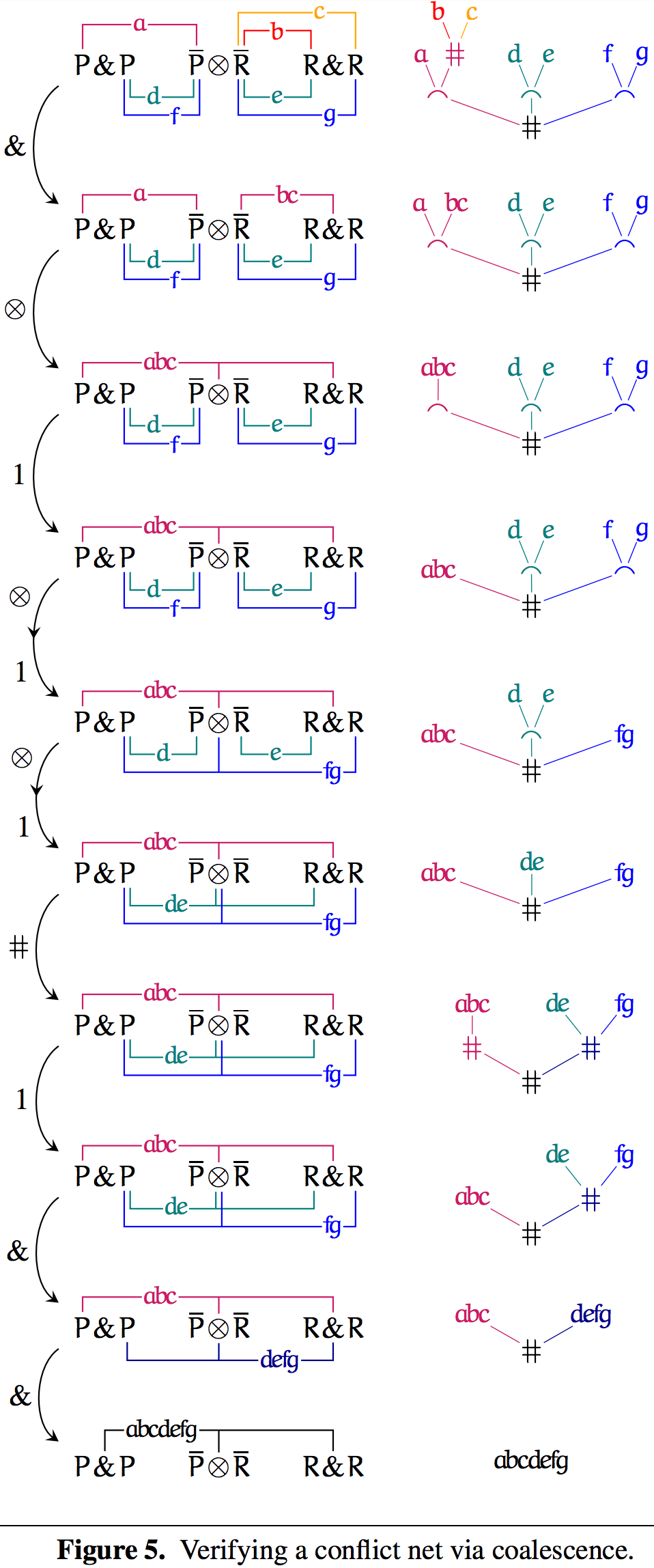### Dominic Hughes - Selected Papers

Conflict nets: Efficient locally canonical MALL proof nets [pdf]
With Willem Heijltjes
Logic in Computer Science, 2016

Proof nets for MLL (unit-free multiplicative linear logic) and ALL (unit-free additive linear logic) are graphical abstractions of proofs which are efficient (proofs translate in linear time) and canonical (invariant under rule commutation). This paper solves a three-decade open problem: are there efficient canonical proof nets for MALL (unit-free multiplicative-additive linear logic)?

Honouring MLL and ALL canonicity, in which all commutations are strictly local proof-tree rewrites, we define local canonicity for MALL: invariance under local rule commutation. We present new proof nets for MALL, called conflict nets, which are both efficient and locally canonical.

Slides from LICS presentation, July 6, 2016.